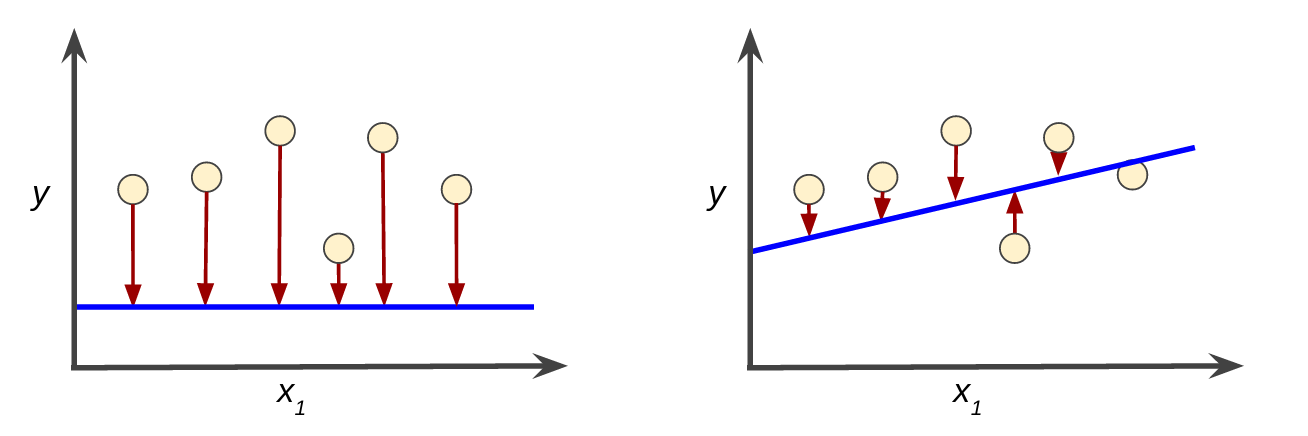Google的《机器学习速成课程 》主要从 机器学习概念机器学习工程机器学习现实世界应用示例 三方面介绍了机器学习。我学完后对速成课程的感受是，对机器学习中的概念解释非常透彻，对机器学习中的部分算法通过动画的方式演示非常直观，概念解释结合示例说明非常丰富，推荐给要学习机器学习的伙伴！

## 问题的构建：机器学习主要术语

### 模型

• 训练 表示创建或学习模型。也就是说，您向模型展示有标签样本，让模型逐渐学习特征与标签之间的关系。
• 推断 表示将训练后的模型应用于无标签样本。也就是说，您使用训练后的模型来做出有用的预测 ($y^{‘}$)。例如，在推断期间，您可以针对新的无标签样本预测。

#### 回归与分类

• 加利福尼亚州一栋房产的价值是多少？
• 用户点击此广告的概率是多少？

• 某个指定电子邮件是垃圾邮件还是非垃圾邮件？
• 这是一张狗、猫还是仓鼠图片

## 训练与损失### 平方损失：一种常见的损失函数

$= \text{the square of the difference between the label and the prediction} \\ = (observation - prediction(x))^{2} \\ = (y - y^{‘})^{2}$

$$MSE=\frac{1}{2}\sum_{(x,y)\in D}(y-prediction(x))^{2}$$

• $(x, y)$ 指的是样本，其中
• $x$ 指的是模型进行预测时使用的特征集（例如，温度、年龄和交配成功率）
• $y$ 指的是样本的标签（例如，每分钟的鸣叫次数）
• $prediction(x)$ 指的是权重与偏差和特征集$x$结合的函数。
• $D$ 指的是包含多个标签样本的数据集
• $N$ 指的是 $D$ 中的样本数量